Courses

# Stress Concentration (Part - 1) Mechanical Engineering Notes | EduRev

## Mechanical Engineering : Stress Concentration (Part - 1) Mechanical Engineering Notes | EduRev

The document Stress Concentration (Part - 1) Mechanical Engineering Notes | EduRev is a part of the Mechanical Engineering Course Machine Design.
All you need of Mechanical Engineering at this link: Mechanical Engineering

Introduction

In developing a machine it is impossible to avoid changes in cross-section, holes, notches, shoulders etc. Some examples are shown in figure- 3.2.1.1.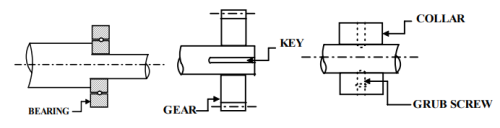3.2.1.1F- Some typical illustrations leading to stress concentration.

Any such discontinuity in a member affects the stress distribution in the neighbourhood and the discontinuity acts as a stress raiser. Consider a plate with a centrally located hole and the plate is subjected to uniform tensile load at the ends. Stress distribution at a section A-A passing through the hole and another

section BB away from the hole are shown in figure- 3.2.1.2. Stress distribution away from the hole is uniform but at AA there is a sharp rise in stress in the vicinity of the hole. Stress concentration factor kt is defined as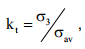where σav at section AA is simply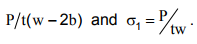This is the theoretical or geometric stress concentration factor and the factor is not affected by the material properties.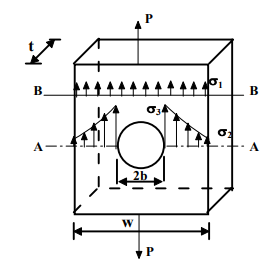3.2.1.2F- Stress concentration due to a central hole in a plate subjected to an uni-axial loading.

It is possible to predict the stress concentration factors for certain geometric shapes using theory of elasticity approach. For example, for an elliptical hole in an infinite plate, subjected to a uniform tensile stress σ(figure- 3.2.1.3), stress distribution around the discontinuity is disturbed and at points remote from the discontinuity the effect is insignificant. According to such an analysis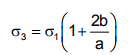If a=b the hole reduces to a circular one and therefore σ3 = 3σ1  which gives kt =3. If, however ‘b’ is large compared to ‘a’ then the stress at the edge of transverse crack is very large and consequently k is also very large. If ‘b’ is small compared to a then the stress at the edge of a longitudinal crack does not rise and kt =1.

'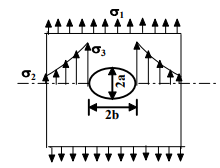3.2.1.3F- Stress concentration due to a central elliptical hole in a plate subjected to a uni-axial loading.

Stress concentration factors may also be obtained using any one of the following experimental techniques:

1. Strain gage method
2. Photoelasticity method
3. Brittle coating technique
4. Grid method

For more accurate estimation numerical methods like Finite element analysis may be employed.

Theoretical stress concentration factors for different configurations are available in handbooks. Some typical plots of theoretical stress concentration factors and  r/d ratio for a stepped shaft are shown in figure-3.2.1.4.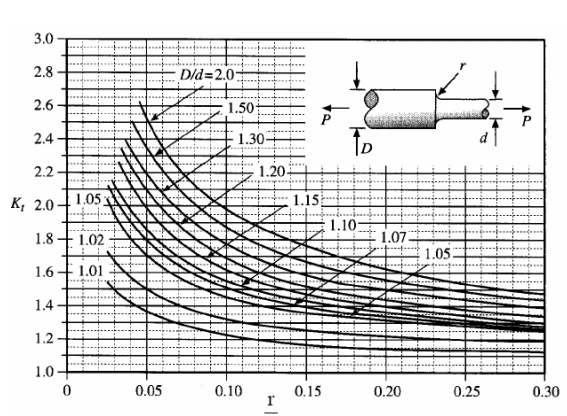3.2.1.4F- Variation of theoretical stress concentration factor with r/d of a stepped shaft for different values of D/d subjected to uni-axial loading .

In design under fatigue loading, stress concentration factor is used in modifying the values of endurance limit while in design under static loading it simply acts as stress modifier. This means Actual stress=kt �calculated stress.

For ductile materials under static loading effect of stress concentration is not very serious but for brittle materials even for static loading it is important.

It is found that some materials are not very sensitive to the existence of notches or discontinuity. In such cases it is not necessary to use the full value of kt and

instead a reduced value is needed. This is given by a factor known as fatigue strength reduction factor kf and this is defined as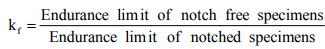Another term called Notch sensitivity factor, q is often used in design and this is defined as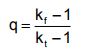The value of ‘q’ usually lies between 0 and 1. If q=0, kf =1 and this indicates no notch sensitivity. If however q=1, then kf = kt and this indicates full notch sensitivity. Design charts for ‘q’ can be found in design hand-books and knowing kt , kf may be obtained. A typical set of notch sensitivity curves for steel is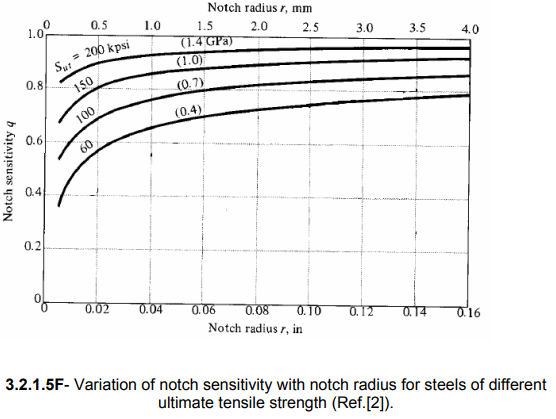Methods of reducing stress concentration

A number of methods are available to reduce stress concentration in machine parts. Some of them are as follows:
1. Provide a fillet radius so that the cross-section may change gradually.
2. Sometimes an elliptical fillet is also used.
3. If a notch is unavoidable it is better to provide a number of small notches rather than a long one. This reduces the stress concentration to a large extent.
4. If a projection is unavoidable from design considerations it is preferable to provide a narrow notch than a wide notch.
5. Stress relieving groove are sometimes provided. These are demonstrated in figure- 3.2.2.1.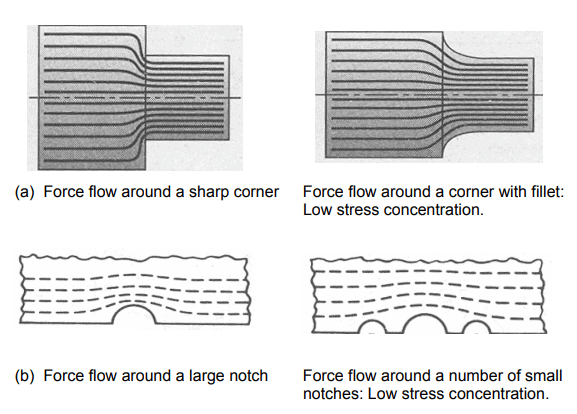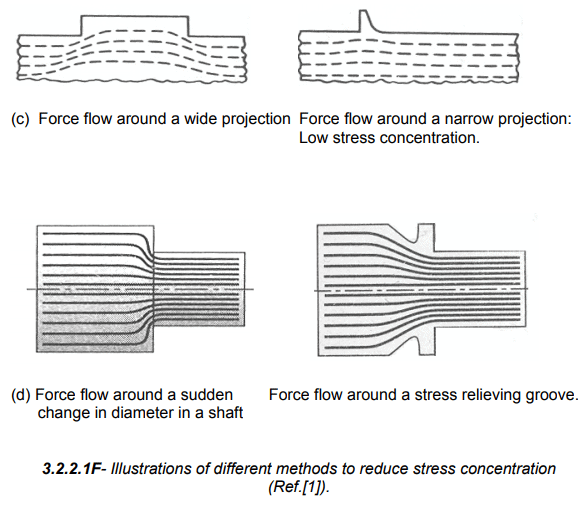Offer running on EduRev: Apply code STAYHOME200 to get INR 200 off on our premium plan EduRev Infinity!

## Machine Design

57 videos|71 docs|102 tests

,

,

,

,

,

,

,

,

,

,

,

,

,

,

,

,

,

,

,

,

,

;